Previous: Model setting without an Up: Water turbines – notes Next: Interface between rotor and
This is an automatically generated documentation by LaTeX2HTML utility. In case of any issue, please, contact us at info@cfdsupport.com.

## Conclusion

We have seen there are two basic approaches to water turbine calculations.
1. With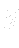in the momentum equation
1. physical setting
2. model setting
2. Without explicitin the momentum equation
Following table shows possible boundary conditions for the pressure variable
Table: Boundary conditions
 pressure setting inlet outlet, physical hTP =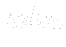fMV =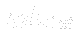, model hTP =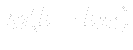fMV =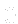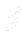hTP =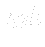fMV =We note that hTP stands for hydrostaticTotalPressure boundary condition and values listed in the Tablefor this type represent values of hydrostatic pressure in the centre of mass of the inlet surface. Whereas fMV stands for fixedMeanValue boundary condition and values listed in the Tablefor this type represent values of static pressure in the centre of mass of the outlet surface. We add that pressureInletVelocity boundary condition is prescribed for the inlet velocity field for all of the above settings, where the velocity magnitude is computed from the difference between total and static pressure and its direction is taken as a local normal to the inlet surface (usually planar). Also zeroGradient boundary condition is prescribed for the outlet surface for all of the above settings.